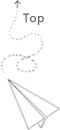# Error of representing numbers

Some tips about representing errors in c/c++

The fact is that the computer can not express all numbers in math. These fact cause some error here and there in the program, we need to be careful about these errors

### Comparing two double or float value

When we try to compare two double or float value, we use abs(a,b) < epsilon to represent it, since they are not totoally same with each other.

### Exceeding the limit

The type of number have their limitation, since there are limited number of byte for each type of data, if we do not care about these issues, the computation can be totoally wrong at some point.

We always need to care about the scope of the input number and if the type of the data we choose can properly express the computed results for numerical operations.

### The point is located in which cell

Sometimes, we need the operation to adjust one point is in which cell, however, the boundry of the cell might be a small number such as 0.000001 which is used to represent 0, then in this case, it is not 0, so when we check the point (0,0) it is not located in the cell, in this case, we need some tolerance value to make the boundry a little bit wider than what it actually is.

Then the followed up question is that, one point can be located in several cells at the same time because of this tolerance issue.

I’m still not sure if the line of cell itsself take up space, but considering one point is located on the cell boundry exactly, we might think it is at the two cells at the same time, especially when we use the tolerance to compare two double value

## 推荐文章